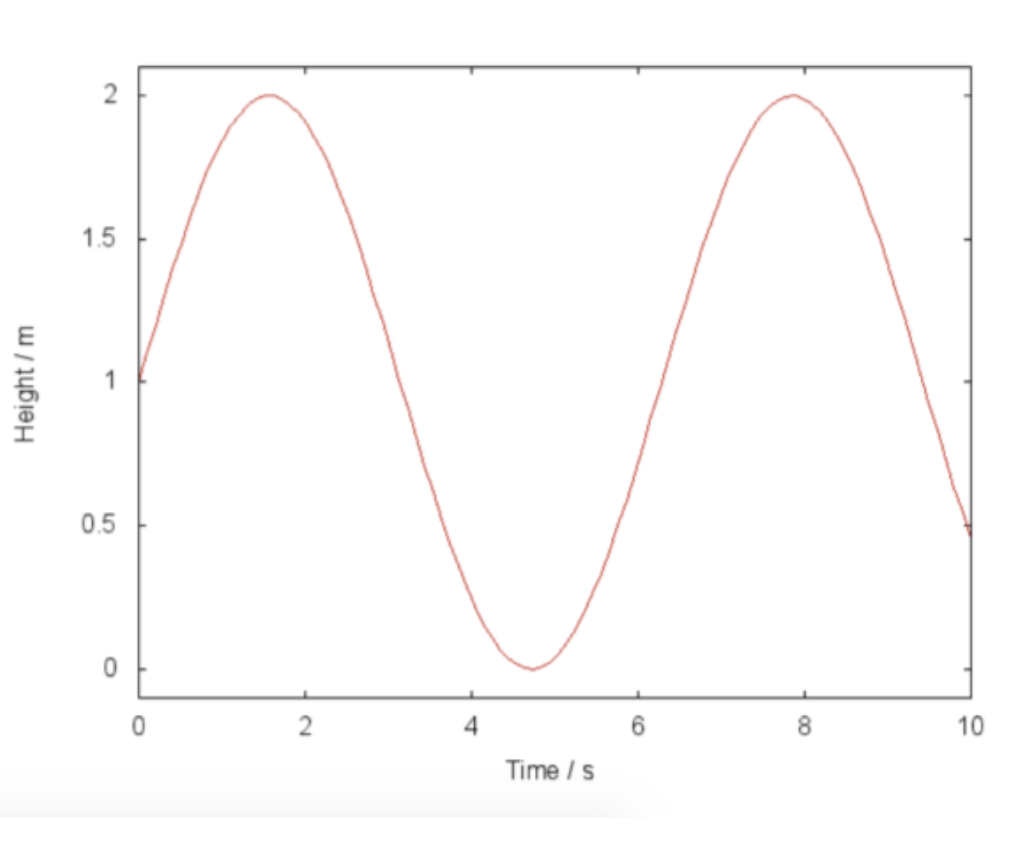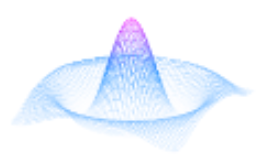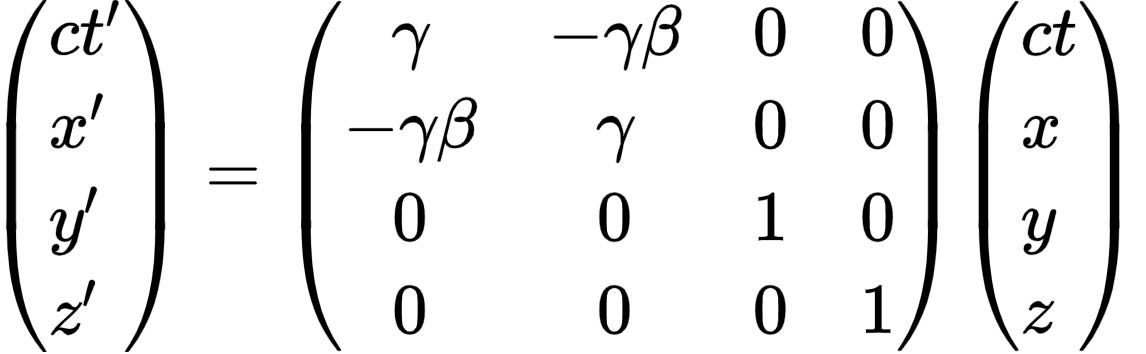# LaTeX Style Figures

To create a figure that floats, use the figure environment:
\begin{figure}[h]
\includegraphics[width=0.5\textwidth, center]{https://cdn.mathpix.com/snip/images/MJT22mwBq-bwqrOYwhrUrVKxO3Xcu4vyHSabfbG8my8.original.fullsize.png}
\end{figure}The \includegraphics[...]{...} command is the one that actually included the image in the document.
[...] contains image size and alignment information;
{...} contains the file path/name (in our case it will contain the image URL)
[width=..., height=..., left] - contains image size and alignment information.
The default alignment for figures is center aligned.
So, if it does not include alignment information, the figure is center aligned (see example below).
If the figure should be left aligned, use [width=..., left], if it should be right aligned, use [width=..., right] (see examples below)
The default size for images is [width=0.5\textwidth] - this means the width of the image will be half the width of the text area, not the page (so not including margin space). Also it supports pt, px, in
Simplest figure syntax:
\begin{figure}
\includegraphics{https://cdn.mathpix.com/snip/images/MJT22mwBq-bwqrOYwhrUrVKxO3Xcu4vyHSabfbG8my8.original.fullsize.png}
\end{figure}Same figure, left aligned:
\begin{figure}
\includegraphics[width=0.5\textwidth, left]{https://cdn.mathpix.com/snip/images/MJT22mwBq-bwqrOYwhrUrVKxO3Xcu4vyHSabfbG8my8.original.fullsize.png}
\end{figure}Same figure, right aligned:
\begin{figure}
\includegraphics[width=0.5\textwidth, right]{https://cdn.mathpix.com/snip/images/MJT22mwBq-bwqrOYwhrUrVKxO3Xcu4vyHSabfbG8my8.original.fullsize.png}
\end{figure}## Captions

It is always a good practice to add a caption to any figure or table. Fortunately, it is very easy in LaTeX. All you need to do is to use the \caption{''text''} command within the float environment. LaTeX will automatically keep track of numbering the figures, so you do not need to include this within the caption text.
The location of the caption is traditionally underneath the float. However, it is up to you to insert the caption command after the actual contents of the float (but still within the environment). If you place it before, then the caption will appear above the float.
The following example demonstrates this effect:
\begin{figure}
\caption{The caption is at the top.}
\centering
\end{figure}

\begin{figure}
\centering
\caption{The caption is at the bottom.}
\end{figure}

\begin{table}
\centering
\begin{tabular}{| l c r |}
\hline
1 & 2 & 3 \\
4 & 5 & 6 \\
7 & 8 & 9 \\
\hline
\end{tabular}
\caption{A simple table}
\end{table}

Notice how the tables and figures have independent counters.

Figure 1: The caption is at the top.Figure 2: The caption is at the bottom.
 1 2 3 4 5 6 7 8 9
Table 1: A simple table
Notice that the tables and figures have independent counters.

## Labels

Figures, just as many other elements in a LaTeX document (equations, tables) can be referenced within the text. It is very easy, just add a label to the figure environment, then later use that label to refer to the picture.
\begin{figure}[h]
\centering
\includegraphics[width=0.25\textwidth]{https://cdn.mathpix.com/snip/images/g1XhFj6GfjHL6KSxW6sPe3vISkV6qk6aCLaEDPbdVZM.original.fullsize.png}
\caption{a nice plot}
\label{fig:mesh1}
\end{figure}

As you can see in the figure \ref{fig:mesh1}
As you can see in the figure \ref{fig:NASA_Logo}.Figure 3: a nice plot
As you can see in the figure 3.
As you can see in the figure 5.

## More examples

\begin{figure}
\includegraphics[width=350px, height=70px, right]{https://cdn.mathpix.com/snip/images/-HM3WXo8kgdk8VtayBtn1_pJlgq9Cb7qV4JtM47Hgn0.original.fullsize.png}
\caption{Equation}
\label{fig:figure1}
\end{figure}Figure 4: Equation
\begin{figure}[H]
\centering